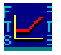Restart to return homeWhat is Vega?

Vega is a number that measures the change in the option value with respect to a change in the volatility of the underlying asset price process.  .

Suppose the vega of a call option equals 17.38 when the volatility of the underlying asset price process is 25% annualized.   This means that if volatility increases by 0.01 (i.e., 0.25 increases to 0.26) then the predicted increase in the option price is 0.01*17.38 = 0.1738.  Thus if the option price is initially 7.488 the changed price is predicted to equal 7.488+0.176= 7.664.

First, why do we care about vega?

Vega gives the option trader insight into how there position value is exposed to volatility.

You may at this stage raise a flag depending upon your prior background experience with traditional option pricing models.  That is, how can we talk about option price exposure to volatility when the Black-Scholes option pricing model and its variations assumes volatility to be constant.

Although this objection has some validity, in an earlier lesson (the implied volatility smile), you can observe first hand that when fitting option pricing model to market price data a constant volatility assumption does not literally hold.

In fact, the assumption of constant volatility across different strike prices and maturities is almost always never confirmed by the data.  Instead both an implied volatility smile (changing volatility with strike prices) and an implied volatility structure (changing volatility with time to maturity) is observed.

Thus on one hand the original objection to the constant volatility assumption has merit.  In practice, however, the constant volatility Black Scholes model is still the most widely applied option pricing model.  This is because different volatility numbers can be entered for different strikes/maturities.  Admittedly this is a bit "ad hoc," but it provides a reasonable practical approximation.  It also provides simple hedge parameters relevant to various risk management support systems.

Estimates of volatility by strike and maturity can be readily obtained from market prices and implied volatility estimates.  Option traders and market makers will often maintain extensive implied volatility charts when trading options.

A Graphical Analysis of Vega

Online, you can now plot vega against asset price.  For an at-the-money IBM option observe that this option price is most sensitive to changes in volatility if the underlying price moves just below the strike price.  This is irrespective of whether you are working with a put or a call option.

In addition, if you plot vega against maturity you can see that the option (irrespective of whether it is a call or a put option) becomes more sensitive to volatility changes as the time to maturity lengthens.  That is, long maturity options, such as LEAPS, are more sensitive to changes in volatility than are short maturity options.

This is a useful property provided by LEAPS compared to stocks.  That is, LEAPS provide an important way of trading volatility.

If you have studied modern portfolio theory you have previously learnt that volatility (or unsystematic firm specific risk) is not predicted to be a driver of a stock price because it is a source of risk that can be freely diversified away by forming a portfolio.  LEAPS on the other hand provide traders with a way of trading volatility.  To gain a complete understanding of the precise economic reasons why this is the case is beyond this lesson's objective.  However, the intuition behind the result is as follows.

Why is Volatility a Major Driver of Option Prices?

If you have already worked through the lessons on delta and gamma you will appreciate that hedging an option's position requires what is referred to as a dynamic hedge as opposed to a static hedge.  A static hedge implies that the hedge is set up once and then left as is over the time the hedge is in place.  A dynamic hedge, on the other hand, requires constant adjustment as the underlying asset price changes.

The major difference between a forward contract (an obligation to exchange at a future point in time, the underlying asset for a price established today) and an option contract (the right to exchange at a future point in time the underlying asset for a contractually specified strike price) is how each contract is hedged.  The forward can be hedged using a static hedge but the option can only be hedged using a dynamic hedge.  The arbitrage free forward price does not depend upon volatility because the cost of carrying the static hedge is independent of volatility.  This is not the case for the arbitrage free option price because now the cost of carrying a dynamic hedge is no longer independent of volatility.

Thus, contrast the problem facing a market maker in forward contracts versus a market maker in an option contract.   Both market makers are in the business of earning the bid/ask spread as opposed to forecasting future price movements.  However, order flows are irregular over time which means that they can never completely off set bids quantities against ask quantities at any point in time.  Thus to earn the spread requires hedging their market making activities against the price risk generated from an irregular order flow.

But now our two market makers face different hedging costs.  The market maker in forwards can hedge and forget (i.e., set up a static hedge).  That is, volatility is irrelevant.   The market maker in options cannot hedge and forget because their hedging problem is dynamic.  As such, volatility is relevant and therefore influences the market maker's pricing of an option.

OS Financial Trading System, PO Box 11356, Pittsburgh, PA 15238 USA, Phone 1-800-967-9897, Fax 1-412-967-5958, Email fts@ftsweb.com,